upm  1.7.1 Sensor/Actuator repository for libmraa (v2.0.0)

## Public Types

}
uint16_t enum containing values for setting gain for ADS1X15 devices.

}
uint16_t enum containing values used for selecting ADS1X15 read operations.

enum  ADSCOMP { CQUE_1CONV = 0x0000, CQUE_2CONV = 0x0001, CQUE_4CONV = 0x0002, CQUE_NONE = 0x0003 }
uint16_t enum containing values for setting ADS1X15 comparator queue modes.

enum  ADSTHRESH { THRESH_LOW = ADS1X15_REG_POINTER_LOWTHRESH, THRESH_HIGH = ADS1X15_REG_POINTER_HITHRESH, CONVERSION_RDY = 0x04, THRESH_DEFAULT = 0x05 }
uint8_t enum containing register addresses used for setting HI and LOW threshold values as well as setting conversion ready and set to default.

enum  ADSSAMPLERATE { SPS_DEFAULT = 0x0080 }
uint16_t enum containing values representing the sample rate of the device. Will be overridden in subclass

## Public Member Functions

std::string name ()

float getLastSample (int reg=ADS1X15_REG_POINTER_CONVERT)

virtual void setSPS (ADSSAMPLERATE rate)

bool getCompMode (void)

void setCompMode (bool mode=false)

bool getCompPol (void)

void setCompPol (bool mode=false)

bool getCompLatch (void)

void setCompLatch (bool mode=false)

bool getContinuous (void)

void setContinuous (bool mode=false)

float getThresh (ADSTHRESH reg=THRESH_DEFAULT)

void setThresh (ADSTHRESH reg=THRESH_DEFAULT, float value=0.0)

## Protected Member Functions

virtual float getMultiplier (void)=0

virtual void setDelay (void)=0

void getCurrentConfig ()

void updateConfigRegister (uint16_t update, bool read=false)

uint16_t swapWord (uint16_t value)

## Protected Attributes

std::string m_name

float m_conversionDelay

uint8_t m_bitShift

uint16_t m_config_reg

mraa::I2c * i2c

## Constructor & Destructor Documentation

 ADS1X15 ( int bus, uint8_t address )

Parameters
 bus i2c bus the sensor is attached to. address Device address. Default is 0x48.
virtual

## Member Function Documentation

 std::string name ( )
inline

Returns the name of the sensor

 float getLastSample ( int reg = `ADS1X15_REG_POINTER_CONVERT` )

Returns the contents of conversion register without performing a conversion operation. Will use a multiplier based on the current gain setting to give the voltage as a float. Used internally to return the HI and LOW threshold values.

Parameters
 reg uint8_t value specifying register to read. Should generally be called with no parameter.

Here is the caller graph for this function:float getSample ( ADSMUXMODE mode = `ADS1X15::DIFF_0_1` )

Performs a read as specified by ADS1X15::ADSMUXMOE and returns the value as a float. Uses getLastSample() internally to return voltage value.

mode ADSMUXMODE specifying inputs to be sampled.

Here is the call graph for this function: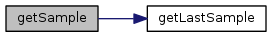Here is the caller graph for this function: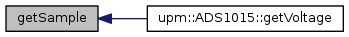ADSGAIN getGain ( )
inline

Returns the current gain setting being used by the device as an ADSGAIN value.

 void setGain ( ADSGAIN gain = `ADS1X15::GAIN_TWO` )

Sets the PGA gain bits to the desired gain. Default is +/- 2.094 volts.

Parameters
 gain ADSGAIN value reprenting the desired gain. See warnings in spec sheet.

Here is the caller graph for this function: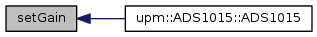ADSSAMPLERATE getSPS ( void )
inline

Returns the current device sample rate a an ADSSAMPLERATE value.

Here is the caller graph for this function: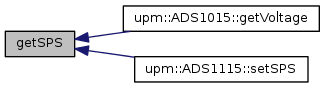void setSPS ( ADSSAMPLERATE rate )
virtual

Sets the sample rate of the device. This function needs to be overrode in subclasses as the ADS1115 and ADS1015 have different data rates.

Parameters
 ADSSAMPLERATE enum SPS_DEFAULT = 0x0080
 bool getCompMode ( void )
inline

Returns the comparator mode. False = Traditional comparator with Hysteresis (default) True = Window Comparator

 void setCompMode ( bool mode = `false` )

Sets the comparator mode of the device.

Parameters
 mode bool value denoting mode. False = Traditional comparator with Hysteresis (default) True = Window Comparator
 bool getCompPol ( void )
inline

Get comparator polarity. Reports the polarity of the ALERT/RDY pin. Returns: False = Active Low (default) True = Active High

 void setCompPol ( bool mode = `false` )

Sets the comparator polarity. Controls the polarity of the ALERT/RDY pin.

Parameters
 mode bool. False = Active Low (default) True = Active High
 bool getCompLatch ( void )
inline

Returns bool representing the state of the comparator latching functionality. False = Non Latching comparator (default) True = Latching Comparator

 void setCompLatch ( bool mode = `false` )

Sets bit controlling comparator operation.

Parameters
 mode bool False = Non Latching comparator (default) True = Latching Comparator
 ADSCOMP getCompQue ( void )
inline

Returns ADSCOMP value representing the state of comparator queue.

CQUE_1CONV = Assert after one conversion CQUE_2CONV = Assert after two conversions CQUE_2CONV = Assert after four conversions CQUE_NONE = Disable comparator (default)

 void setCompQue ( ADSCOMP mode = `ADS1X15::CQUE_NONE` )

Sets bits controlling Comparator queue operation.

Parameters
 mode ADSCOMP enum. CQUE_1CONV = Assert after one conversion CQUE_2CONV = Assert after two conversions CQUE_2CONV = Assert after four conversions CQUE_NONE = Disable comparator (default)
 bool getContinuous ( void )
inline

Returns bool reflecting state of device mode bit.

False = Power Down Single shot mode (default) True = Continuous conversion mode

 void setContinuous ( bool mode = `false` )

Sets the state of device mode but.

Parameters
 mode bool False = Power Down Single shot mode (default) True = Continuous conversion mode
 float getThresh ( ADSTHRESH reg = `THRESH_DEFAULT` )

Returns current high or low threshold setting.

Parameters
 reg ADSTHRES enum value. Returns 0.0 unless THRESH_HIGH or THRESH_LOW requested.

Here is the call graph for this function: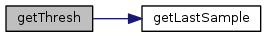void setThresh ( ADSTHRESH reg = `THRESH_DEFAULT`, float value = `0.0` )

Sets threshold levels or configures for conversion ready operation of ALERT/RDY output.

Parameters
 reg ADSTHRESH enum value float value to set threshold register to.

THRESH_LOW = Sets low thresh register. THRESH_HIGH = Sets high thresh register. CONVERSION_RDY = Configures conversion ready operation THRESH_DEFAULT = resets high/low registers to startup values.

Inheritance diagram for ADS1X15: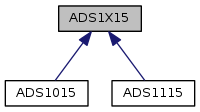[legend]

The documentation for this class was generated from the following files: